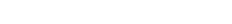Categories

# Marginalizing out Normal-Wishart Prior of multivariate Normal distribution for Bayesian inference

I found my old notes, and writing this post based on the notes for record.

The goal of this particular note is to marginalize out the parameters of multivariate Normal distribution using Normal-Wishart conjugate prior. This is useful for Bayesian inference for Gaussian distribution.

First of all, let me derive the joint distribution of observation as follows,where D is the number of dimensions and Z is the partition function of Wishart distribution, i.e.,andNow, it is ready to marginalize out the mu and omega. The marginal distribution isI have omitted the detail of the derivation of (2) from (1). But, the following equations are useful to derive (2) from (1)